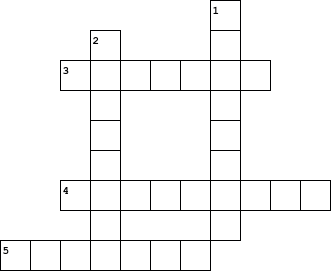You can also copy (CTRL a CTRL c) and paste (CTRL v) this into Microsoft Word to customize it.

## Math TermsAcross 3. number being divided by 4. part left after dividing 5. answer in a multiplication problem

 Down 1. answer in division problem 2. number being divided